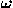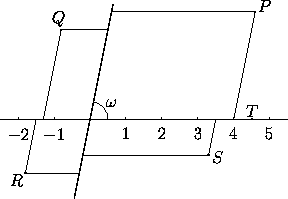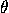# 1.5 Oblique Coordinates in the Plane

The following generalization of cartesian coordinates is sometimes useful. Consider two axes (graduated lines), intersecting at the origin but not necessarily perperdicularly. Let the angle between them be. In this system of oblique coordinates, a point P is given by two real numbers indicating the positions of the projections from the point to each axis, in the direction of the other axis. See Figure 1. The first axis (x-axis) is generally drawn horizontally. The case=90° yields a cartesian coordinate system.Figure 1: In oblique coordinates, P=(4,3), Q=(-1.3,2.5), R=(-1.5,-1.5), S=(3.5,-1), and T=(4.5,0). Compare Figure 1.2.1 .

### Relations between two oblique coordinate systems

Let the two oblique coordinate systems (x,y) and (x',y'), with anglesand', share the same origin, and suppose the x'-axis makes an anglewith the x-axis. The coordinates (x,y) and (x',y') of a point in the two systems are related by

x = (x' sin(-) + y' sin(-'-))/ sin,
y = (x' sin+ y' sin('+))/ sin.

This formula also covers passing from a cartesian system to an oblique system and vice versa, by taking=90° or'=90°.

The relation between two oblique coordinate systems that differ by a translation is the same as for cartesian systems: see (1.1.4) .

Next: 2 Plane Symmetries or Isometries
Up: 1 Coordinate Systems in the Plane
Previous: 1.4 Homogeneous Coordinates in the PlaneThe Geometry Center Home Page

Silvio Levy
Wed Oct 4 16:41:25 PDT 1995

This document is excerpted from the 30th Edition of the CRC Standard Mathematical Tables and Formulas (CRC Press). Unauthorized duplication is forbidden.